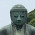## 2011/11/02

### How much does "Beta" change depending on time?

You may often use "Beta" to measure the market exposure of your portfolio because it's easy to calculate.
Since I have been wondering how much "Beta" change depending on time, more precisely writing, data-set and the period of return time series, I think that I would like to write about that in this article.

First, I get stock price( I selected  Mitsubishi UFJ Financial Group, Inc here) and market index(Nikkei225 ) from yahoo Japan by using RFinanceYJ package. These data period are from September, 2010 to September, 2011.
```library(RFinanceYJ)
#download stock prices of nikkei-225 and MUFJ Financial group
mufg   <- quoteStockXtsData("8306.T",   since="2010-09-30",date.end="2011-09-30")\$Close
nikkei <- quoteStockXtsData("998407.O", since="2010-09-30",date.end="2011-09-30")\$Close```
(you need to install RFinanceYJ Package to run this code)

I convert this price data into return data, and estimate the "Beta" of  Mitsubishi UFJ Financial Group, Inc by using rolling linear regression(every regression have 125 sample data).

```#convert to return
returns <- merge(mufg   / lag(mufg) - 1, nikkei / lag(nikkei) - 1)
names(returns) <- c("MUFG","NIKKEI")
#estimate beta by rolling regression
size.window <- 125
coefs <- rollapplyr(returns, size.window, function(x)coef(lm(x[,1]~x[,2])), by.column = FALSE)```
As you know, R language provides us a very easy way to analyze, That is it.
So, Let's visualize these result. The result of simple plot is following that.

`plot(as.xts(coefs[, 2]))`

This graph shows that how historical beta changes depending on time.
And I create the animation of this result to understand more easily.

As you find, The result of linear regression strongly depends on the period of data set!
If we want to get a more robust result, we should you use something like "robust" regression :D
Here is the code to create this animation.

```x <- na.omit(coredata(returns)[ ,2])
y <- na.omit(coredata(returns)[ ,1])
x.max <- c(-max(abs(x)), max(abs(x)))
y.max <- c(-max(abs(y)), max(abs(y)))
x.lab <- names(returns)
y.lab <- names(returns)
Snap <- function(val){
val.x <- na.omit(coredata(val)[ ,2])
val.y <- na.omit(coredata(val)[ ,1])
lm.xy <- lm(val.y~val.x)
plot(val.x, val.y, xlim = x.max, ylim = y.max,
xlab = x.lab, ylab = y.lab)
abline(lm.xy)
text(x.max, y.max, paste("Beta :", round(coef(lm.xy),3)), pos = 4)
text(x.max, y.max, as.character(last(index(val))), pos = 4)
}

library(animation)
saveGIF({
for(i in 1:(nrow(returns)-size.window)){
Snap(returns[(i:(i+size.window)),])
}
},interval = 0.01)```
you need to install "animation" package and ImageMagick (another software) to run this code

Enjoy!

```
```

#### 2 件のコメント:

1.Sorry, a Lazy question, would we be able to get a stock from another exchange (say London, via yahoo, say if I want the stock GSK.L, how would I query with the function quoteStockXtsData)

Thanks,

Athula

2.Thank you for your comment!

RFinanceYJ package gives only Japanese stock market data to you.

If you want to get another exchange data, you should use quandmod package.(http://www.quantmod.com/documentation/getSymbols.yahoo.html)